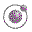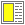Name binding, Recursion, Iteration, and Continuations - slide 8 : 42

LAML time functions

 Expression Value `(current-time)` `999789132` `(time-decode 1000000000)` `(2001 9 9 3 46 40)` `(time-decode 0)` `(1970 1 1 2 0 0)` `(time-interval 1000000000)` `(31 8 2 5 1 46 40)` `(weekday (current-time))` `Thursday` `(danish-week-number (current-time))` `36`